Get instant live expert help with Excel or Google Sheets“My Excelchat expert helped me in less than 20 minutes, saving me what would have been 5 hours of work!”

#### Post your problem and you’ll get expert help in seconds.

Your message must be at least 40 characters
Our professional experts are available now. Your privacy is guaranteed.

# How to Insert Current Date and Time from your System in Google Sheets and Excel through a Formula

Excel offers some very important functions for date and times. To get the current date and time we can use the NOW and TODAY functions. In this tutorial, we will learn how to insert current date and time in Excel.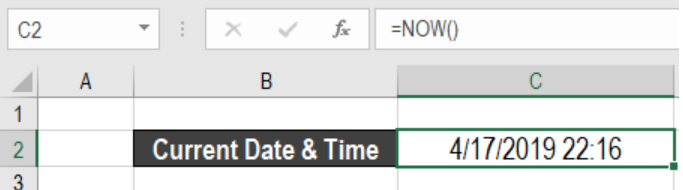Figure 1. Current Date and Time in Excel

## How to Insert Current Date and time in Excel

To get the current date and time from our system in Excel, we can use the NOW() function. This function updates the time and date every time the worksheet is opened.

### Syntax

`=NOW()`

The NOW function does not have any arguments. It shows the current date and time from our system. Every time we open or recalculate the worksheet, NOW updates the date and time.

To show the current date and time in cell C2, we can use the formula `=NOW()`. This shows the current date and time in cell C2.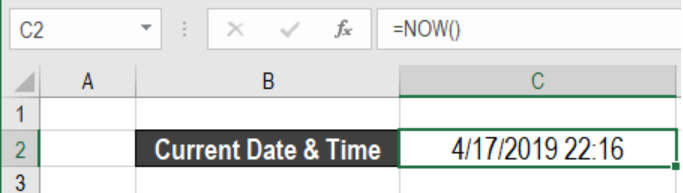Figure 2. Example of How to Insert Current Date and Time in Excel

## How to Insert Date in Excel

If we want to display the date only, we can change the date format. We can also use the TODAY function. It shows the date from our system.

### Syntax

`=TODAY()`

TODAY in Excel also works like the NOW function. It only displays the date and updates every time the worksheet is opened. To display the current date in cell C3, we use the formula `=TODAY()`.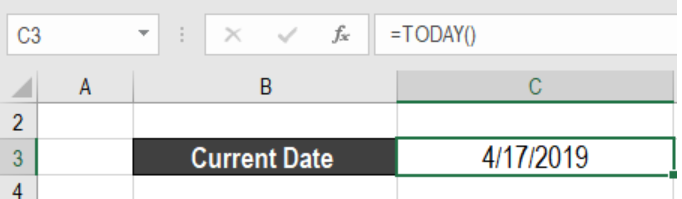Figure 3. Example of How to Insert Date in Excel that Updates Every Time

## How to Insert Current Time in Excel

If we need to insert the current time in a way that will not change, use the keyboard shortcut Ctrl + Shift + ;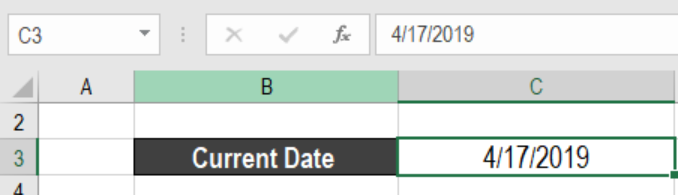Figure 4. Example of How to Insert Date in Excel that Does not Update

This shortcut will insert the current time in a cell. This value will not change automatically. To insert both the date and time in a cell, we need to use the keyboard shortcut Ctrl + ; Next, we have to enter a space character and press Ctrl + Shift + ;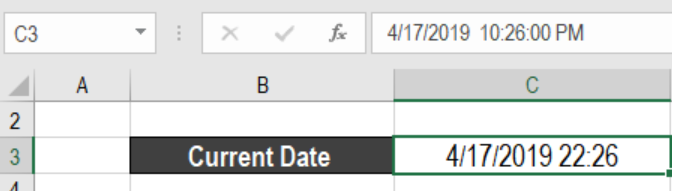Figure 5. Example of How to Insert Current Date and Time in Excel that Does not Update

## How to Insert Current Date and Time from your System in Google Sheets

We can insert the current date and time in Google Sheets using the NOW and TODAY functions.

To insert the current date and time in Google Sheets, we need to

• Go to cell B2.
• Assign the formula `=NOW()`.
• Press Enter.

This will show the current date and time in Google Sheets.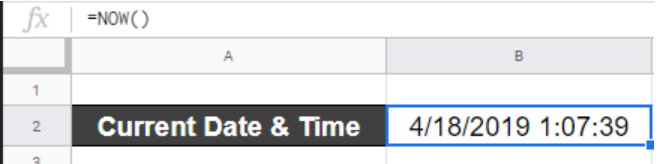Figure 6. Example of How to Insert Current Date and Time in Google Sheets

By using the TODAY function, we only get the current date in Excel. To show the current date in cell B2, we need to

• Insert a function in cell B2 to display the current date from our system. This would be the function `=TODAY()`.
• Press Enter.

This will show the current date in Google Sheets.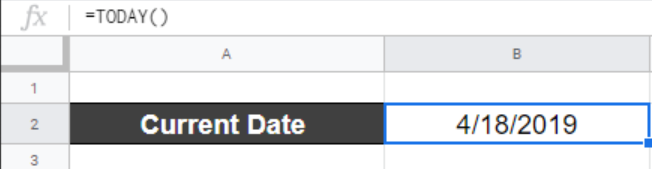Figure 7. Example of How to Insert Date in Google Sheets

The date updates every time we open or recalculate the worksheet.

Most of the time, the problem you will need to solve will be more complex than a simple application of a formula or function. If you want to save hours of research and frustration, try our live Excelchat service! Our Excel Experts are available 24/7 to answer any Excel question you may have. We guarantee a connection within 30 seconds and a customized solution within 20 minutes.

Solution examplesI would need a formula what copies from my DataInput sheet into another one daily the first two column A and B.
Solved by K. S. in 33 minsI would need a formula what copies from my DataInput sheet into another one daily the first two column A and B.
Solved by B. C. in 60 minsI need the formula for time accrual to auto populate in E4 based on certain criteria. example: B4 = hire date E4= accrual rate If todays date is equal to or greater than 60 days but less than 1 year from B4 date then E4 = 1 If todays date is equal to or greater than 3 years but less than 4 years from B4 date then E4 = 1.08 If todays date is equal to or greater than 5 years but less than 9 years from B4 date then E4 = 1.5 If todays date is equal to or greater than 10 years but less than 14 years from B4 date then E4 = 1.58 If todays date is equal to or greater than 15 years from B4 date then E4 = 1.92
Solved by A. H. in 60 minsI would like my result cell to display 1 of 5 values: If my referenced cell value is less than 2 then the result cell should display 2. (values 0 thru 1.99 = 2) but if my referenced cell value is greater than 2 but less than 2.99 then the new cell should display 3 (values 2 thru 2.99 = 3) but if my referenced cell value is greater than 3 but less than 4.99 then the new cell should display 5 (values 3 thru 4.99 = 5) but if my referenced cell value is greater than 5 but less than 7.5 then the new cell should display 7.5 (values 5 thru 7.5 = 7.5)
Solved by S. Q. in 15 minsi want to insert a multiplication formula in a cell if some percentage value is entered in another cell of same row. for eg. if i put a formula in G7 cell (=G2*e7) but it should be enabled or activated if i put some data entry in f7 cell Excel
Solved by Z. D. in 20 mins